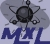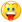## Welcome to the Second Life Forums Archive

These forums are CLOSED. Please visit the new forums HERE

# Bulk integer storage / quasi-pointers

Madox Kobayashi
Madox Labs R&DJoin date: 28 Jun 2003
Posts: 402
08-10-2003 06:32
Ever need a crap load of integer variables in a script but you found that: you hit the heap collision error? refering to a ton of variables by name in impractical or just wont work?

I recently needed >200 variables and I needed to refer to them programatically somehow, not by name. So here is a useful funtion to let you all do that.

value() uses a rotation variable to store 16 2-digit integers. You can adjust to script to store 8 4-digit integers or 32 1-digit integers.

Variables 0-3 are stored in the x coordinate, 4-7 in the y coordinate, 8-11 in z, 12-15 in s. This sample code uses 2 rotations, so variables 16-31 are in the second rotation.

I was able to store my 200 variables in 13 rotations, saving a lot of memory.

Enjoy!

Usage:
integer value(integer set, integer index, integer v)

set - TRUE is you are setting a variable, FALSE if retrieving
index - the variable number to get/set
v - the value to set. ignored if set is FALSE

returns 0 if set is TRUE.
return the value if variable at index if set is FALSE.

CODE
`rotation a;rotation b;integervalue(integer set, integer index, integer v) {    rotation rot;    float fl;    float i = llFloor(index/16.0);    float j = llFloor((index-(i*16))/4.0);    float k = (index-(i*16)) - (j*4);        if (i == 0) rot = a;    else if (i == 1) rot = b;        if (j == 0) fl = rot.x;    if (j == 1) fl = rot.y;    if (j == 2) fl = rot.z;    if (j == 3) fl = rot.s;    if (set == FALSE) {        float fl2 = llFloor(fl / llPow(100,k));        float fl3 = fl2 - llFloor(fl2/100.0)*100.0;        return (integer)fl3;    } else {        float existing = llFloor(fl / llPow(100,k));        existing = existing - llFloor(existing/100.0)*100.0;        fl = fl - (existing * llPow(100,k));        fl = fl + (v * llPow(100,k));        if (j == 0) rot.x = fl;        if (j == 1) rot.y = fl;        if (j == 2) rot.z = fl;        if (j == 3) rot.s = fl;        if (i == 0) a = rot;        else if (i == 1) b = rot;        return 0;    }}`
_____________________
Madox Kobayashi

Madox Kobayashi
Madox Labs R&DJoin date: 28 Jun 2003
Posts: 402
08-13-2003 17:13
As you are all learning, I'm hideously bad at explaining and teaching programmingI posted this because I'm sure it will help avoid stack/heap collisions, so for you all, and Derek, here is what it is doingI have made a maze generator object recently. You all will see it in action soon when Ironchef and I unveil it. It is a 10x10 cel maze that gets created randomly - and so I needed 100 variables to store wall info for each cel.

I first did it with a big list, but to update a value I had to delete a sublist and insert another sublist. This used a lot of memory and it only generated 40 cels before hitting stack/heap.

To get rid of lists I could use 100 variables, but there would be no way to loop over them at all. There are no arrays to use. I would need a gigantic if/else thing.

But by making all me data numeric, and making it so I only needed values 0-99, I could use a float to hold 4 variables.

float x = 40302010;

x is storing 4 variables that have the values 10, 20, 30 and 40.

I then used rotations because they have 4 floats in them and will make for more compact code. So a single rotation can hold 16 of my variables. I can get 112 variables from 7 rotations.

This example only uses 2 rotations so it holds 32 variables.

To see each variable, here is the 16 varaibles being initialized to the values 1 through 16:

rotation a = <04030201, 08070605, 12111009, 16151413>;

So in the code, you pass in INDEX which is the variable number. Index can be from 0 to15. That has to be converted into rotation number, rotation member and location in that float (which of the 4 locations)

i is the rotation number (rotation a is #1, rotation b is #2)
j is the member number (x is #1... s is #4)
k is the location in the float (44332211)

float fl is set equal to the resulting float containing 4 variables, one of which is the one you are refering to with index.

If you are retieving (set = FALSE) the value of your variable is extracted and returned. Example of extracting the 2nd variable from 40302010:

fl = 40302010
fl = 40302010 / 100 = 403020
fl = 403020 - 403000 = 20
fl = 20 which gets returned

If you are saving (set = TRUE) the opposite happens and your value you passed in gets saved into fl which gets saved back into the proper rotation member.

Using this I was able to get about 90-95 cels generated and code pruning got me the rest of the way. It was no slower than using lists, which goes to show how fast lists are.

Hope that made sense.
You can add more rotations and even use 4 digit numbers instead of 2 if you have to, but each float can only hold 2 variables then.

The other upshot is that you know have a kind of pointer, because you can address each variable by its 'address', the index, and not have to pass around values.
_____________________
Madox Kobayashi